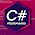## Sunday, May 19, 2013

### Operators Precedence in C#

Operator precedence determines the grouping of terms in an expression. This affects how an expression is evaluated. Certain operators have higher precedence than others; for example, the multiplication operator has higher precedence than the addition operator:
For example x = 7 + 3 * 2; Here x is assigned 13, not 20 because operator * has higher precedence than + so it first get multiplied with 3*2 and then adds into 7.
Here operators with the highest precedence appear at the top of the table, those with the lowest appear at the bottom. Within an expression, higher precedence operators will be evaluated first.
Category Operator Associativity
Postfix () [] -> . ++ - -  Left to right
Unary + - ! ~ ++ - - (type)* & sizeof Right to left
Multiplicative  * / % Left to right
Additive  + - Left to right
Shift  << >> Left to right
Relational  < <= > >= Left to right
Equality  == != Left to right
Bitwise AND Left to right
Bitwise XOR Left to right
Bitwise OR Left to right
Logical AND && Left to right
Logical OR || Left to right
Conditional ?: Right to left
Assignment = += -= *= /= %=>>= <<= &= ^= |= Right to left
Comma Left to right

1.2.3.